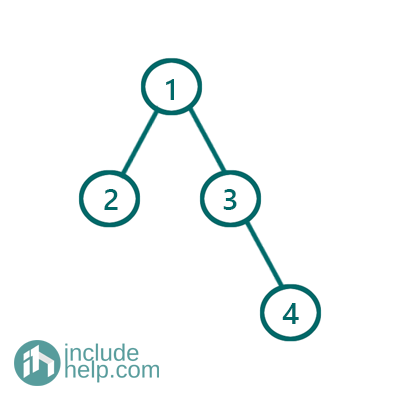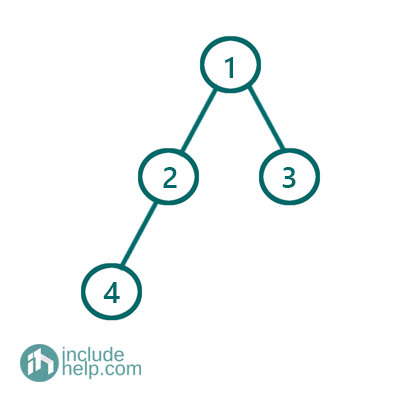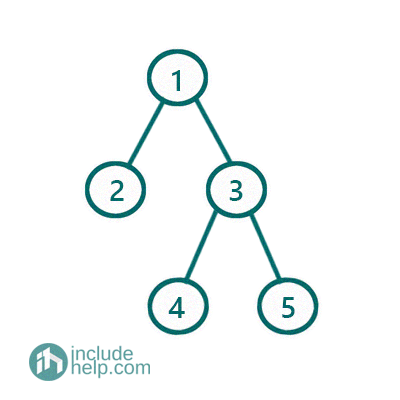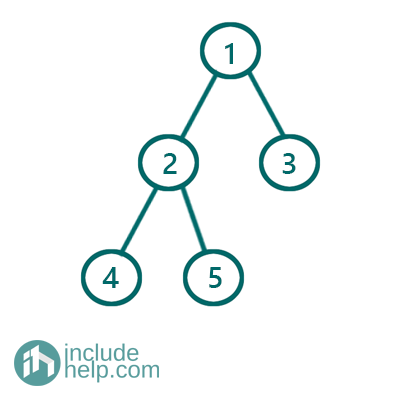# Complete Binary Tree

In this article, we are going to see what Complete Binary Tree is and what are the properties of a complete binary tree and differences between Full Binary Tree and Complete Binary Tree. Also, in the end, we have added a Program to compute the number of nodes in a complete binary search efficiently using its properties.
Submitted by Radib Kar, on July 31, 2020

Prerequisite:

In the last article we talked about full binary tree, and complete binary tree is another classification of binary tree. As per Defined in Wikipedia:

In a complete binary tree every level, except possibly the last, is completely filled, and all nodes in the last level are as far left as possible. A complete Binary Tree can have between 1 and 2h nodes inclusive at the last level h.

So, the properties of complete Binary tree are:

1. All levels are filled up except the last level
2. The nodes at the last levels are as far left as possible

## Examples of Complete Binary Tree

Below are a few examples where we have showed which one is Full Binary Tree and which is Complete Binary Tree. There are cases also where a binary tree is both full and complete and also there are cases where a binary tree is neither a full binary tree nor a complete binary tree.

### 1) Neither Complete Binary Tree Nor Full Binary TreeFigure 1: Neither Full nor complete binary tree

The above binary tree is neither a complete Tree nor a Full Binary Tree.

It's not a Full Binary Tree because the node with value 3 has only one children. The tree is not complete because the node at the last level is not as left as far possible.

### 2) Complete Binary Tree but not Full Binary TreeFigure 2: Complete Binary Tree but not Full Binary Tree

The above Tree is not a Full Binary Tree because the node with value 2 has only one child. But the above tree is complete as the node at the last level is as left as far possible and other levels are completely filled.

### 3) Full Binary Tree but not Complete Binary treeFigure 3: Full Binary Tree but Not complete binary tree

The above tree is a Full binary tree has each node has either two or zero children. But it's not a complete binary tree as the nodes at the last level is not as much left as far possible.

### 4) Both Full Binary Tree and Complete Binary TreeFigure 4: Both Full & Complete Binary Tree

The above tree is both Complete and Full binary tree as it follows both the protocols. Like, all nodes have either zero or two children which makes it a full binary tree & the last level has nodes as left as far possible, and all the previous levels are completed which makes it a complete binary Tree.

Hope, the above examples clears all confusion b/w complete and full binary tree and also helps to distinguish between them.

## Application of complete Binary Tree

We have a very vast application of complete binary tree which is heap

## Properties of Complete Binary tree and counting number of nodes efficiently

As per definition the complete binary tree has two properties:

1. All the levels are completed except the last levels
2. The last level has leaf nodes as left as far possible.

So, we can count total number of nodes using these two properties:

Say, total number of nodes is n

And the height of the complete binary tree is: h

Then it's guaranteed that all levels up to height h-1 is completed which means has 2h-1 number of nodes up to the last level. At the last level say there r k number of nodes which are as left as much possible. So, we total no of nodes n= k+ 2h-1

So, we need to find two things:

1. Height of the tree
2. Number of nodes at the last level

Height of the tree can be found in O(Log2n) time and we can find k also in logarithmic time complexity using the fact that all the nodes at last level lies as left as far possible.

The algorithm is like below:

1. If root is NULL return 0
2. Compute the height of the tree, h.
3. If height is 1 then return 1( Only root is there)
4. As we discussed earlier that all the levels except the last one completed and has 2h-1 number of nodes. We can compute that in constant time as we already know h. Now, we have to calculate number of nodes at the last level, k. The number of nodes in the last level can be anything between 1 to 2h. Now to find out exact number of nodes, we perform the binary search like the below implementation by the node index to check how many nodes are in the last level. Say the function is is_exists which takes number of nodes we expect to be at last level, height of tree and returns true or false. It returns true if the last level has same more nodes that we expected and returns false if the last node has less number of notes than expected. We can find this also in logarithmic time as shown as in the implementation(It's possible because of the fact that all nodes are as left as far possible in the last level for complete binary tree). So overall complexity for finding number of nodes is O(logn)*O(logn)

So over all time complexity is O(logn) (to find height and number of nodes in all levels except the last one)+O(logn)2 (to find number of nodes in the last level)

C++ Implementation:

```#include <bits/stdc++.h>
using namespace std;

class TreeNode {
public:
int val;
TreeNode* left;
TreeNode* right;

TreeNode(int v)
{
val = v;
left = NULL;
right = NULL;
}
};

bool is_exists(TreeNode* root, int h, int no_of_nodes)
{
TreeNode* cur = root;

int left = 0, right = pow(2, h) - 1;
//loop runs for h=O(logn) time
//binary search to find the no_of_nodes th
//node exist or not
for (int i = 0; i < h; ++i) {
int mid = left + (right - left) / 2;
if (no_of_nodes <= mid) {
cur = cur->left;
right = mid;
}
else {
cur = cur->right;
left = mid + 1;
}
}
//if no_of_nodes at last level exists then
//cur will point to that no_of_nodes th node
//else NULL
return cur != NULL;
}

//since it's a complete tree we can
//traverse towards the left child always
//since the last level nodes will be as
//left as far possible
int height(TreeNode* root)
{
if (!root)
return 0;
TreeNode* cur = root;
int count = 0;
while (cur) {
count++;
cur = cur->left;
}
return count;
}

//calculate number of nodes in the
//complete tree efficiently
int countNodes(TreeNode* root)
{
if (!root)
return 0;
if (!root->left && !root->right)
return 1;

int h = height(root) - 1;
//number of nodes except the last level
//is calculated as follow
int no_of_nodes_except_last_level = pow(2, h) - 1;
//number of possible nodes at the last level
int left = 1, right = no_of_nodes_except_last_level;

int last_level_nodes;
//do binary search to check if predicted number
//of node exists or not
while (left <= right) {
int mid = left + (right - left) / 2;
//finds if last level has mid number of
//nodes or not
if (is_exists(root, h, mid)) {
left = mid + 1;
}
else
right = mid - 1;
}
last_level_nodes = left;
return no_of_nodes_except_last_level + last_level_nodes;
}

int main()
{
cout << "Tree which is both complete and full in example 4\n";

TreeNode* root = new TreeNode(1);

root->left = new TreeNode(2);
root->right = new TreeNode(3);
root->left->left = new TreeNode(4);
root->left->right = new TreeNode(5);

cout << "Number of nodes in this tree: " << countNodes(root) << endl;

return 0;
}
```

Output:

```Tree which is both complete and full in example 4
Number of nodes in this tree: 5
```

Relevant post: Count Number of Nodes in a complete binary tree

What's New

Top Interview Coding Problems/Challenges!

Languages: » C » C++ » C++ STL » Java » Data Structure » C#.Net » Android » Kotlin » SQL
Web Technologies: » PHP » Python » JavaScript » CSS » Ajax » Node.js » Web programming/HTML
Solved programs: » C » C++ » DS » Java » C#
Aptitude que. & ans.: » C » C++ » Java » DBMS
Interview que. & ans.: » C » Embedded C » Java » SEO » HR
CS Subjects: » CS Basics » O.S. » Networks » DBMS » Embedded Systems » Cloud Computing
» Machine learning » CS Organizations » Linux » DOS
More: » Articles » Puzzles » News/Updates